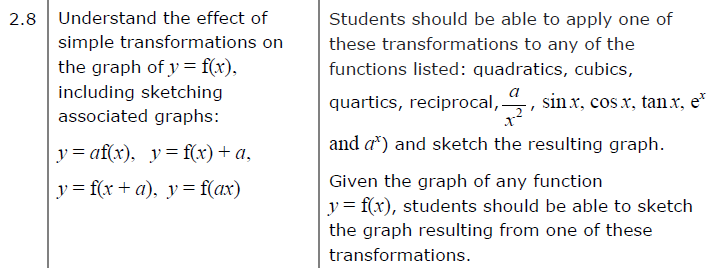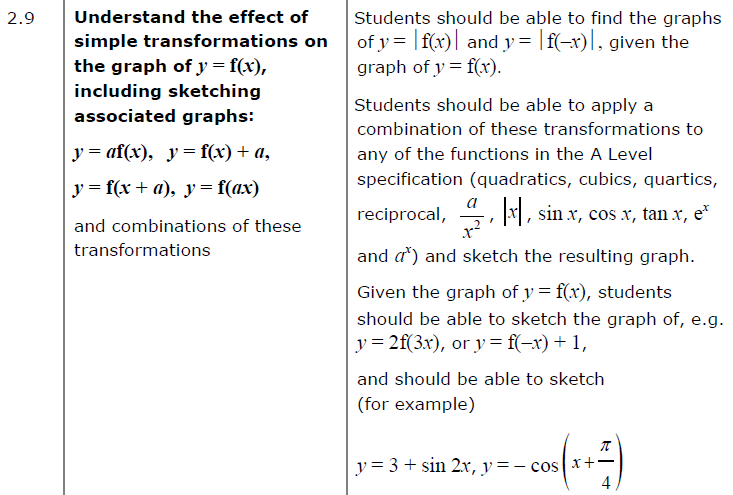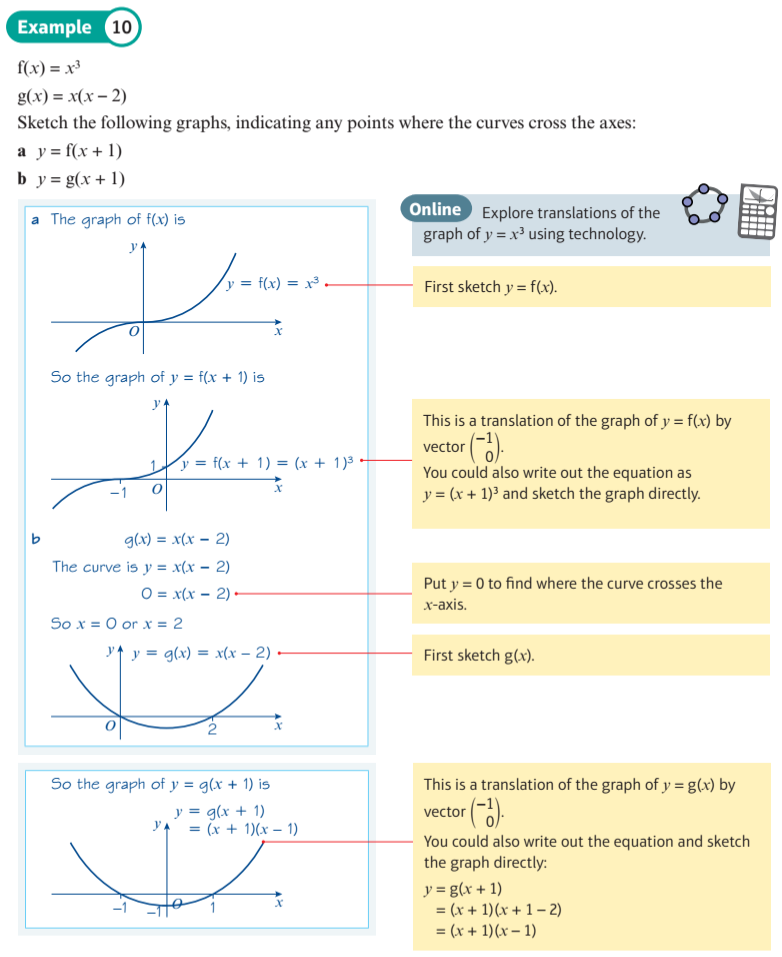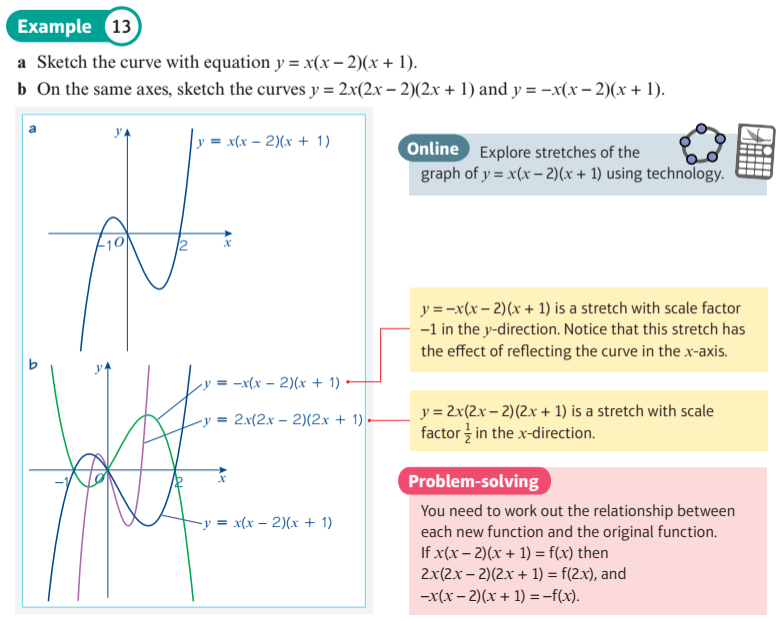Instant Maths Help:

# Edexcel A Level

## Transformations##### Explore translations of a graph##### Explore stretches of a cubic graph##### Transformation: y = a f(x)
For each example, investigate the effect on the graph of y = af(x) of changing 'a'.
A transformation may look like a rotation, but the draggable green point, shows they are all, in fact, stretches away from the x-axis by a factor of a.
What is the effect of making a = -1, so y = -f(x) ?
##### Transformation: y = f(x + a)
For each example, investigate the effect on the graph of y = f(x + a) of changing 'a'.
The green sample points show how graphs are translated horizontally in the opposite direction to what you might expect.
##### Transformation: y = f(x - a)
For each example, investigate the effect on the graph of y = f(x - a) of changing 'a'.
The green sample points show how graphs are translated horizontally.
##### Transformation: y = f(x) + a
For each example, investigate the effect on the graph of y = f(x) + a of changing 'a'.
You can drag the green point, and then use the slider to see the effect of the transformation on that particular point.
##### Transformation: y = f(a x)
For each example, investigate the effect on the graph of y = f(ax) of changing 'a'.
The green sample points show how graphs expand or contract horizontally in the opposite directions to what you might expect.
What is the effect of making a = -1, so y = f(-x) ?
##### Exponential and Log Combined Transformations
Two transformations of $$y = f\left( x \right)$$ are considered: $$y = f\left( {ax} \right)$$ and $$y = f\left( {x - b} \right)$$ , together with their combination.

This display shows the transformation $$y = \ln \left( {ax} \right)$$ What is the effect of increasing $$a$$? It should be a stretch factor $${1 \over a}$$, but it looks like a translation. Noting that $$\ln \left( {ax} \right) = \ln \left( x \right) + \ln \left( a \right)$$, you can see that the transformation is the same as a translation vertically by $$y = \ln \left( {a} \right)$$. When $$a = - 1$$, the transformation is a reflection in the $$y$$ axis.

This display shows the transformation $$y = \ln \left( {x - b} \right)$$ Increasing the value of $$a$$ will move the curve to the right.

This display shows the transformations $$y = \ln \left( {{ax} - b} \right)$$
##### a sin (b x + c) + d Transformations
This display allows you to view transformations of the $\color{blue}{ \sin }$ function.

The original sin function is showed as a green dashed line, and the transformed sin function is shown as a solid blue line. Initially the lines coincide. You can drag green and blue gliders to read coordinates at corresponding points on the original and transformed curves.
##### a cos (b x + c) + d Transformations
This display allows you to view transformations of the $\color{blue}{ \cos }$ function.

The original cos function is showed as a green dashed line, and the transformed cos function is shown as a solid blue line. Initially the lines coincide. You can drag green and blue gliders to read coordinates at corresponding points on the original and transformed curves.
##### a tan (b x + c) + d Transformations
This display allows you to view transformations of the $\color{blue}{ \tan }$ function.

The original tan function is showed as a green dashed line, and the transformed tan function is shown as a solid blue line. Initially the lines coincide. You can drag green and blue gliders to read coordinates at corresponding points on the original and transformed curves.
##### Investigate Order of Transformations 1
This display shows the order in which transformations are applied generally makes a difference.
For $\color{blue}{f(x) = ( a \, \sin ( x ) ) + d }$ the vertical stretch (factor $\color{purple}{ a }$) is applied before the vertical translation (of $\color{purple}{ d }$).
For $\color{red}{g(x) = a \, ( \sin ( x ) + d ) }$ the vertical translation (of $\color{purple}{ d }$) is applied before the vertical stretch (factor $\color{purple}{ a }$).

You can drag a glider on the x-axis to help read cooordinates at corresponding sample points.
##### Investigate Order of Transformations 2
This display shows the order in which transformations are applied generally makes a difference.
For $\color{blue}{f(x) = \sin ( ( b \, x ) + c ) }$ the horizontal translation (of $\color{purple}{ - c }$) is applied before the horizontal stretch (factor $\color{purple}{ { 1 \over b } }$).
For $\color{red}{g(x) = \sin ( b ( x + c ) ) }$ the the horizontal stretch (factor $\color{purple}{ { 1 \over b } }$) is applied before the horizontal translation (of $\color{purple}{ - c }$).
These orders may seem counter-intuitive if considering 'BIDMAS' precedence.

You can drag a glider on the x-axis to help read cooordinates at corresponding sample points.